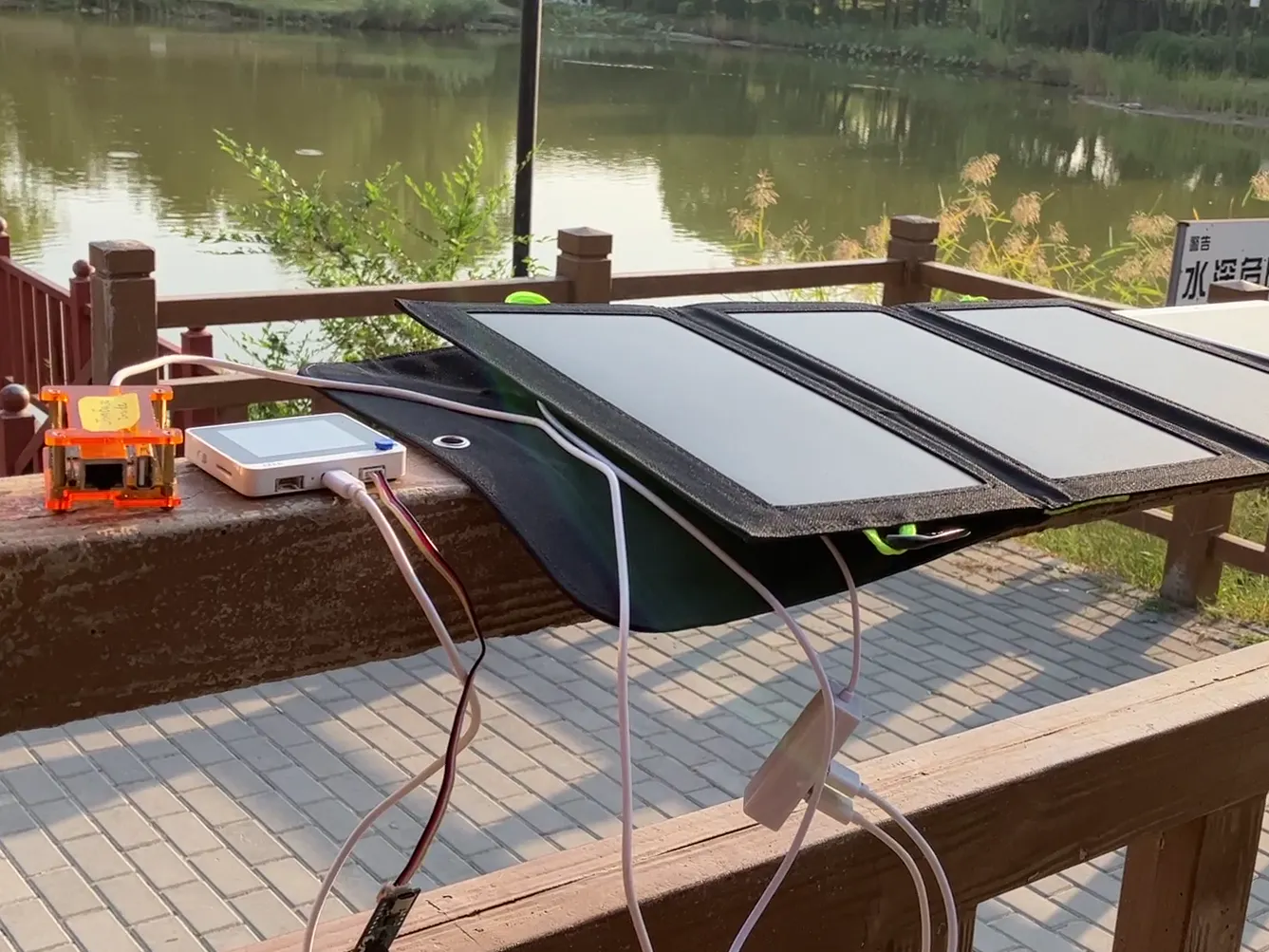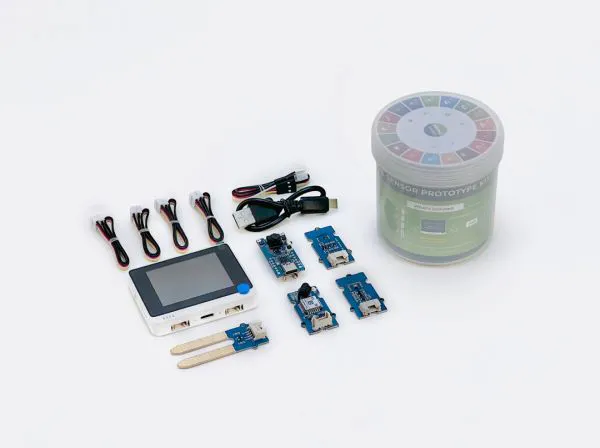Created September 27, 2022

# TinyWild, Make Wild IoT in Your Hand

TinyWild, a low cost on-the-edge realtime data stack for wildlife survey, makes flexible edge IoT data stack in book-sized green space.

IntermediateFull instructions provided2## Things used in this project

### Hardware componentsSeeed Studio SenseCAP K1100 - The Sensor Prototype Kit with LoRa® and AI
×1
 Rock Pi S
×1

### Software apps and online services

 JoinBase

## Code

### animals.py

Python
the script is use to preprocess the animals-80 dataset's labeling to YoLOv5 labeling format
```from ctypes import cdll
import numpy as np  # linear algebra
import pandas as pd  # data processing, CSV file I/O (e.g. pd.read_csv)
import glob
from tqdm import tqdm
import os
import cv2
import matplotlib.pyplot as plt

data_dir = "/iot_in_the_wild/yolo/animal/archive"
train_dir = os.path.join(data_dir, "train")
test_dir = os.path.join(data_dir, "test")

all_train_subdir = glob.glob(train_dir+"/*")
all_test_subdir = glob.glob(test_dir+"/*")

train_classes = [os.path.basename(pp) for pp in all_train_subdir]
test_classes = [os.path.basename(pp) for pp in all_test_subdir]

print("There is %d classes in train dataset, and %d classes in test dataset" %
(len(train_classes), len(test_classes)))

print(train_classes == test_classes)

train_image_counts = {os.path.basename(
pp): [len(glob.glob(os.path.join(pp, "*.jpg")))] for pp in all_train_subdir}
test_image_counts = {os.path.basename(
pp): [len(glob.glob(os.path.join(pp, "*.jpg")))] for pp in all_test_subdir}
# all_image_counts=train_image_counts.copy()
# all_image_counts={k:all_image_counts[k]+test_image_counts[k] for k in all_image_counts.keys()}
train_data_df = pd.DataFrame(train_image_counts, index=["train"]).transpose()
test_data_df = pd.DataFrame(test_image_counts, index=["test"]).transpose()
all_data_df = train_data_df.copy()
all_data_df["test"] = test_data_df

all_data_df = all_data_df.sort_values(by=["train", "test"], ascending=False)

yolo_train_dir = "yolo2/train"
yolo_test_dir = "yolo2/test"

for dd in [yolo_train_dir, yolo_test_dir]:
for ss in ["images", "labels"]:
print(os.path.join(dd, ss))
os.makedirs(os.path.join(dd, ss), exist_ok=True)

for subdir_id in tqdm(range(len(all_train_subdir))):
subdir = all_train_subdir[subdir_id]

def process_dataset(subdirs, dst_dir, class_names, size=(640, 640), link=False):
for subdir_id in tqdm(range(len(subdirs))):
subdir = subdirs[subdir_id]
prefix = os.path.basename(subdir)
for image_file in glob.glob(os.path.join(subdir, "*.jpg")):
image_file_basename = os.path.basename(image_file)
label_file = os.path.join(
subdir, "Label", image_file_basename).replace(".jpg", ".txt")
dst_image_file = os.path.join(
dst_dir, "images/%s_%s" % (prefix, image_file_basename))
dst_label_file = os.path.join(
dst_dir, "labels/%s_%s" % (prefix, image_file_basename.replace(".jpg", ".txt")))
if os.path.exists(dst_label_file):
continue

height, width = image.shape[0:2]
with open(label_file) as fobj:
with open(dst_label_file, "w") as wobj:
while True:
if item is None or len(item) == 0:
break
class_name = prefix
item = item[len(class_name):]
item = item.split()
xmin = float(item)
ymin = float(item)
xmax = float(item)
ymax = float(item)

cx = (xmin + xmax)/2.0/width
cy = (ymin + ymax)/2.0/height
bw = (xmax - xmin)/width
bh = (ymax - ymin)/height
class_id = class_names.index(class_name)
output_line = "%d %f %f %f %f\n" % (
class_id, cx, cy, bw, bh)
wobj.write(output_line)

else:
image = cv2.resize(image, size)
cv2.imwrite(dst_image_file, image)

# process_dataset(all_train_subdir, yolo_train_dir, train_classes, size=(640,640), link=False)
# train_subdir = all_train_subdir[0:1]
train_subdir = all_train_subdir[:]
classes = [os.path.basename(pp) for pp in train_subdir]

print("classes:")
print(classes)

process_dataset(train_subdir, yolo_train_dir,

test_subdir = all_test_subdir[:]
classes = [os.path.basename(pp) for pp in test_subdir]
process_dataset(test_subdir, yolo_test_dir,

yaml_file = "yolov5/data/animal.yaml"
train_images_dir = os.path.join("..", yolo_train_dir, "images")
val_images_dir = os.path.join("..", yolo_test_dir, "images")

names_str = ""
for item in classes:
names_str = names_str + ", \'%s\'" % item
names_str = "names: ["+names_str[1:]+"]"

with open(yaml_file, "w") as wobj:
wobj.write("train: %s\n" % train_images_dir)
wobj.write("val: %s\n" % val_images_dir)
wobj.write("nc: %d\n" % len(classes))
wobj.write(names_str+"\n")
```